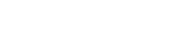# math.exp2

`FLOATmath.exp2FLOATx`

Available inall subroutines.

Computes the value of 2 raised to the `x` power (e.g., 2ˣ).

This is equivalent to shifting the integer 1 to `x` bits to the left (e.g., `2^x == 1 << x`), except that `math.exp2` is only for `FLOAT` type values.

## Example

``vcl_deliver {  declare local var.foo FLOAT;  set var.foo = math.exp2(32); # result: 4294967296.0}``

BETA